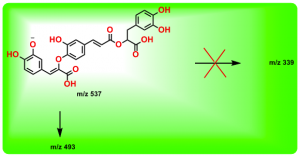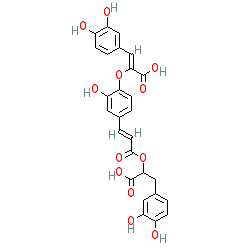SMILES: OC(C(CC1=CC=C(C(O)=C1)O)OC(/C=C/C2=CC=C(C(O)=C2)O/C(C(O)=O)=C\C3=CC(O)=C(C=C3)O)=O)=O
InChIKey: MZSGWZGPESCJAN-JKXXRSRDSA-N

–EOF–